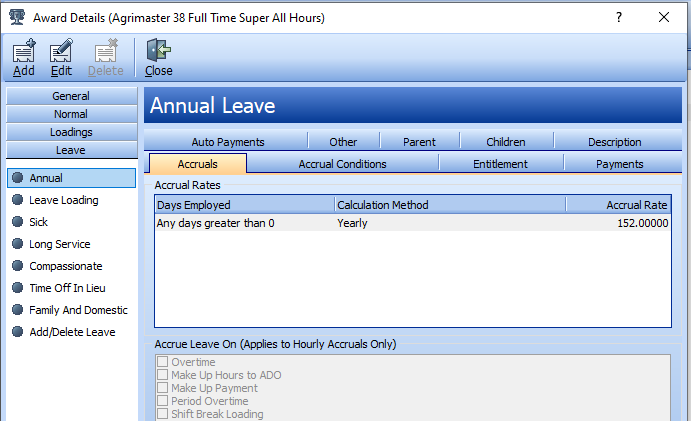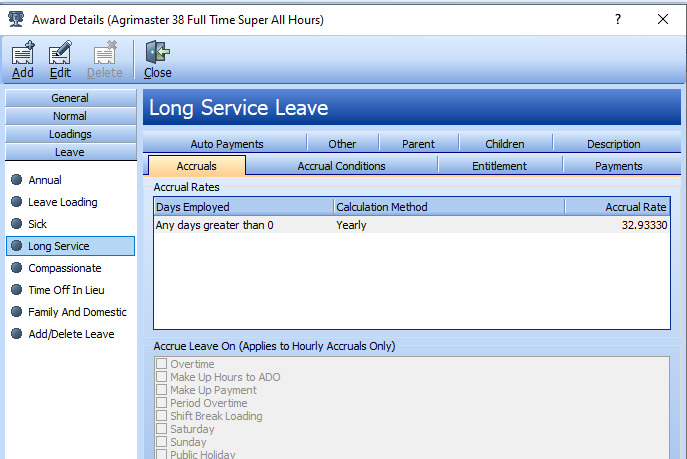Follow

# Calculate the Daily Rate Of Accrual For Each Leave Type

When conducting a reconciliation of your employees' accrued leave records the daily rate of annual leave, personal leave and long service leave (if applicable) must be calculated.

1. Calculate The Daily Rate Of Accrual For Annual Leave
Example
Employee is working 38 hours per week and gets 4 weeks of annual leave per year (38 x 4 = 152.00 hours).
Accrued Annual Leave rate- 152 divided by 365 = 0.4164383 per day. This means that Wagemaster is incrementing this decimal every day. You can repeat the steps for 40 hours per week with 160 hours/year.2. Click on Personal Leave > Accruals tab Calculate The Daily Rate Of Accrual For Personal Leave
This was known as Sick Leave prior to the introduction Fair Work Act.
Example
Employee is working 38 hours per week and receives 2 weeks of personal leave per year (38 x 2 = 76.00 hours).
Accrued Personal Leave rate - 76 weeks divided by 365 = 0.2082191 per day. This means that Wagemaster is incrementing this decimal every day.3. Click on the Long Service Accruals tab. Calculate The Daily Rate Of Accrual For Long Service Leave
Example
The employee is working 38 hours per week and receives 8 2/3 weeks leave after 10-year service. (Dependant State's Long Service Leave Legalisation).
Accrued Long Service Leave - (38 * 8 2/3) = 329.333333 hours divided by 10 to get the accrual rate per year = 32.9333. This means that Wagemaster is incrementing by (32.9333 / 365) = 0.09023 hours every day.